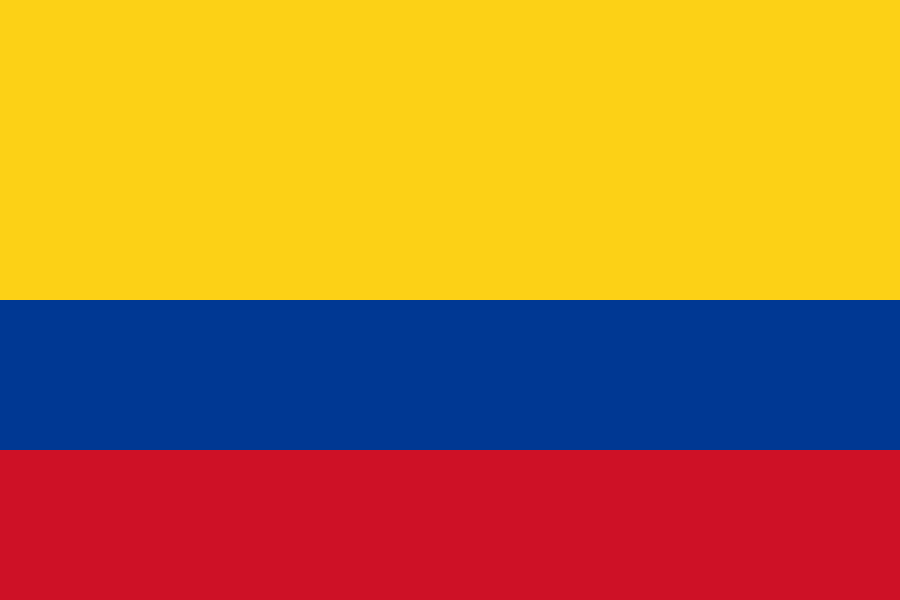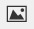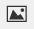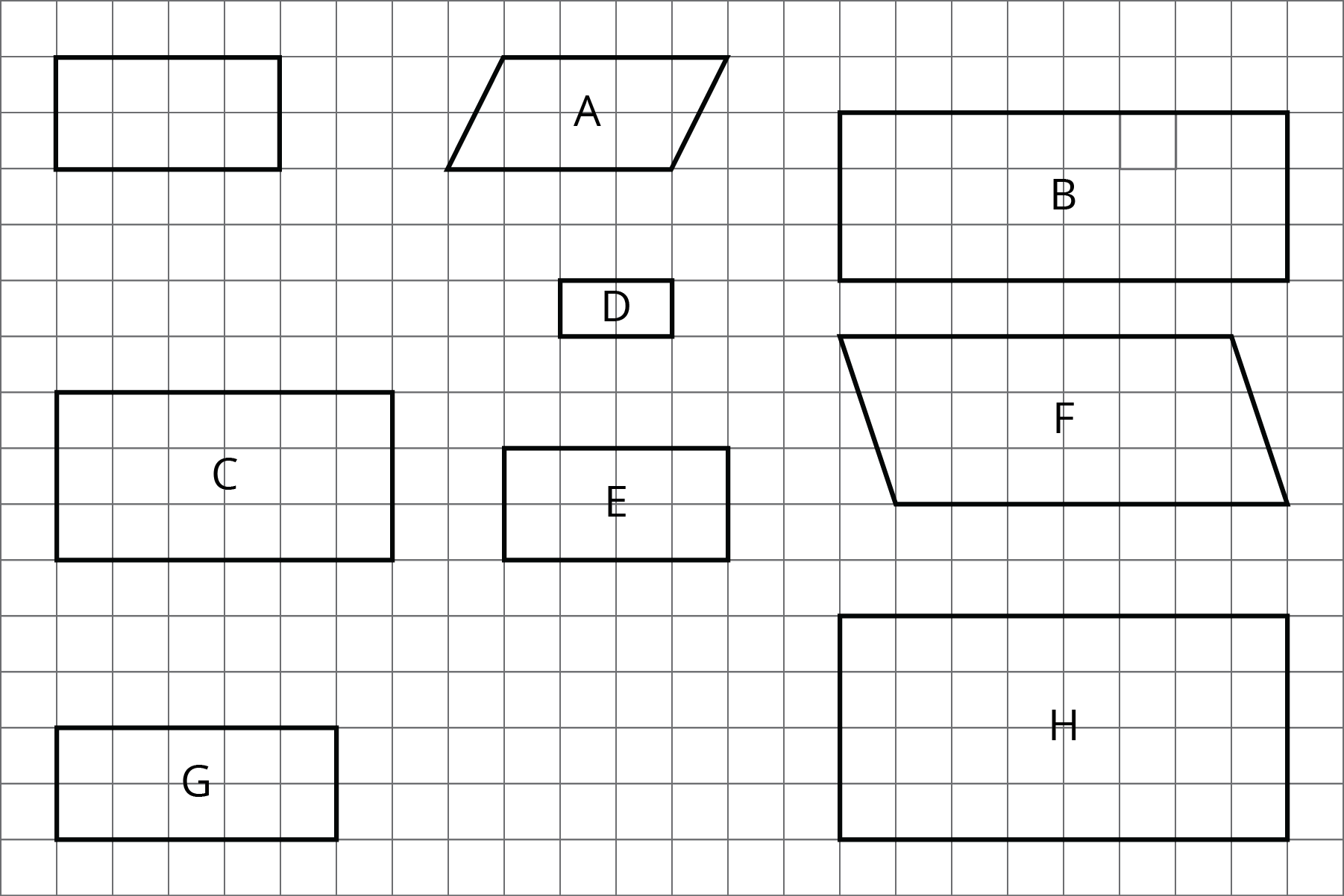PSA6QCV - Practice Problems---7.1 Lesson 9: Creating Scale Drawings (7.G.A.1)
Part A)

An image of a book shown on a website is 1.5 inches wide and 3 inches tall on a computer monitor. The actual book is 9 inches wide.

What scale is being used for the image?

___:___

Part B)

How tall is the actual book?

Type your answer below as a number (example: 5, 3.1, 4 1/2, or 3/2):
Part A)

The flag of Colombia is a rectangle that is 6 ft long with three horizontal strips.• The top stripe is 2 ft tall and is yellow.
• The middle stripe is 1 ft tall and is blue.
• The bottom stripe is also 1 ft tall and is red.

Create a scale drawing of the Colombian flag with a scale of 1 cm to 2 ft.

Draw your drawing on paper, take a picture, and upload it using the image upload iconIf you do not have the ability to upload an image of your work type "Drawing is on paper."

Part B)

Create a scale drawing of the Colombian flag with a scale of 2 cm to 1 ft.

Draw your drawing on paper, take a picture, and upload it using the image upload iconIf you do not have the ability to upload an image of your work type "Drawing is on paper."

Part A)

These triangles are scaled copies of each other.For each pair of triangles listed, the area of the second triangle is how many times larger than the area of the first?

Triangle G and Triangle F

Type your answer below as a number (example: 5, 3.1, 4 1/2, or 3/2):
Part B)

Triangle G and Triangle B

Type your answer below as a number (example: 5, 3.1, 4 1/2, or 3/2):
Part C)

Triangle B and Triangle F

Type your answer below as a number (example: 5, 3.1, 4 1/2, or 3/2):
Part D)

Triangle F and Triangle H

Type your answer below as a number (example: 5, 3.1, 4 1/2, or 3/2):
Part E)

Triangle G and Triangle H

Type your answer below as a number (example: 5, 3.1, 4 1/2, or 3/2):
Part F)

Triangle H and Triangle B

Type your answer below as a number (example: 5, 3.1, 4 1/2, or 3/2):
Part A)
Here is an unlabeled rectangle, followed by other quadrilaterals that are labeled.Select all quadrilaterals that are scaled copies of the unlabeled rectangle.

Select all that apply:
Part B)
Here is an unlabeled rectangle, followed by other quadrilaterals that are labeled.Select all quadrilaterals that are scaled copies of the unlabeled rectangle.

Explain how you know.

Draw your scaled version on paper, take a picture, and upload it using the image upload icon